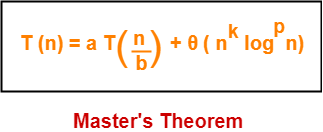## Master Theorem-

 Master’s Theorem is a popular method for solving the recurrence relations.

Master’s theorem solves recurrence relations of the form-Here, a >= 1, b > 1, k >= 0 and p is a real number.

## Master Theorem Cases-

To solve recurrence relations using Master’s theorem, we compare a with bk.

Then, we follow the following cases-

### Case-01:

If a > bk, then T(n) = θ (nlogba)

### Case-02:

If a = band

• If p < -1, then T(n) = θ (nlogba)
• If p = -1, then T(n) = θ (nlogba.log2n)
• If p > -1, then T(n) = θ (nlogba.logp+1n)

### Case-03:

If a < band

• If p < 0,  then T(n) = O (nk)
• If p >= 0, then T(n) = θ (nklogpn)

## Problem-01:

Solve the following recurrence relation using Master’s theorem-

T(n) = 3T(n/2) + n2

## Solution-

We compare the given recurrence relation with T(n) = aT(n/b) + θ (nklogpn).

Then, we have-

a = 3

b = 2

k = 2

p = 0

Now, a = 3 and bk = 22 = 4.

Clearly, a < bk.

Since p = 0, so we have-

T(n) = θ (nklogpn)

T(n) = θ (n2log0n)

Thus,

 T(n) = θ (n2)

## Problem-02:

Solve the following recurrence relation using Master’s theorem-

T(n) = 2T(n/2) + nlogn

## Solution-

We compare the given recurrence relation with T(n) = aT(n/b) + θ (nklogpn).

Then, we have-

a = 2

b = 2

k = 1

p = 1

Now, a = 2 and bk = 21 = 2.

Clearly, a = bk.

Since p = 1, so we have-

T(n) = θ (nlogba.logp+1n)

T(n) = θ (nlog22.log1+1n)

Thus,

 T(n) = θ (nlog2n)

## Problem-03:

Solve the following recurrence relation using Master’s theorem-

T(n) = 2T(n/4) + n0.51

## Solution-

We compare the given recurrence relation with T(n) = aT(n/b) + θ (nklogpn).

Then, we have-

a = 2

b = 4

k = 0.51

p = 0

Now, a = 2 and bk = 40.51 = 2.0279.

Clearly, a < bk.

Since p = 0, so we have-

T(n) = θ (nklogpn)

T(n) = θ (n0.51log0n)

Thus,

 T(n) = θ (n0.51)

## Problem-04:

Solve the following recurrence relation using Master’s theorem-

T(n) = √2T(n/2) + logn

## Solution-

We compare the given recurrence relation with T(n) = aT(n/b) + θ (nklogpn).

Then, we have-

a = √2

b = 2

k = 0

p = 1

Now, a = √2 = 1.414 and bk = 20 = 1.

Clearly, a > bk.

So, we have-

T(n) = θ (nlogba)

T(n) = θ (nlog2√2)

T(n) = θ (n1/2)

Thus,

 T(n) = θ (√n)

## Problem-05:

Solve the following recurrence relation using Master’s theorem-

T(n) = 8T(n/4) – n2logn

## Solution-

• The given recurrence relation does not correspond to the general form of Master’s theorem.
• So, it can not be solved using Master’s theorem.

## Problem-06:

Solve the following recurrence relation using Master’s theorem-

T(n) = 3T(n/3) + n/2

## Solution-

• We write the given recurrence relation as T(n) = 3T(n/3) + n.
• This is because in the general form, we have θ for function f(n) which hides constants in it.
• Now, we can easily apply Master’s theorem.

We compare the given recurrence relation with T(n) = aT(n/b) + θ (nklogpn).

Then, we have-

a = 3

b = 3

k = 1

p = 0

Now, a = 3 and bk = 31 = 3.

Clearly, a = bk.

Since p = 0, so we have-

T(n) = θ (nlogba.logp+1n)

T(n) = θ (nlog33.log0+1n)

T(n) = θ (n1.log1n)

Thus,

 T(n) = θ (nlogn)

## Problem-07:

Form a recurrence relation for the following code and solve it using Master’s theorem-

```A(n)
{
if(n<=1)
return 1;
else
return A(√n);
}```

## Solution-

• We write a recurrence relation for the given code as T(n) = T(n) + 1.
• Here 1 = Constant time taken for comparing and returning the value.
• We can not directly apply Master’s Theorem on this recurrence relation.
• This is because it does not correspond to the general form of Master’s theorem.
• However, we can modify and bring it in the general form to apply Master’s theorem.

Let-

n = 2m    ……(1)

Then-

T(2m) = T(2m/2) + 1

Now, let T(2m) = S(m), then T(2m/2) = S(m/2)

So, we have-

S(m) = S(m/2) +1

Now, we can easily apply Master’s Theorem.

We compare the given recurrence relation with S(m) = aS(m/b) + θ (mklogpm).

Then, we have-

a = 1

b = 2

k = 0

p = 0

Now, a = 1 and bk = 20 = 1.

Clearly, a = bk.

Since p = 0, so we have-

S(m) = θ (mlogba.logp+1m)

S(m) = θ (mlog21.log0+1m)

S(m) = θ (m0.log1m)

Thus,

S(m) = θ(logm)    ……(2)

Now,

• From (1), we have n = 2m.
• So, logn = mlog2 which implies m = log2n.

Substituting in (2), we get-

S(m) = θ(loglog2n)

Or

 T(n) = θ (loglog2n)

To gain better understanding about Master’s Theorem,

Watch this Video Lecture

Next Article- Recursion Tree

Get more notes and other study material of Design and Analysis of Algorithms.

Watch video lectures by visiting our YouTube channel LearnVidFun.